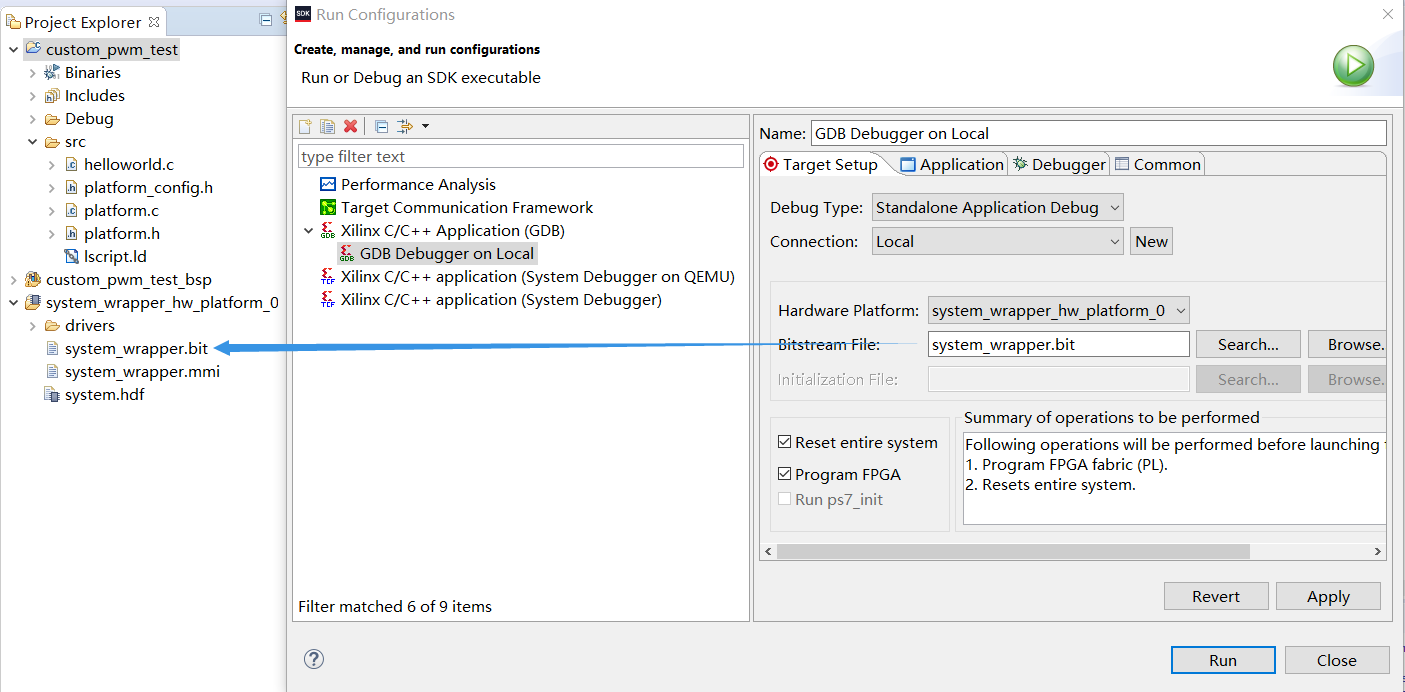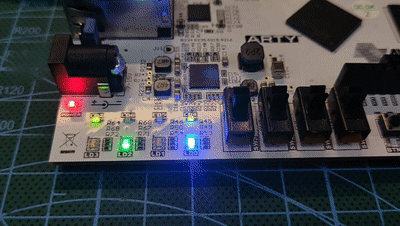# 【Arty-A7填坑笔记】03：为Microblaze定制AXI总线接口的PWM外设

## 0.前言

### 0.2.资源链接

http://www.alientek.com/

Xilinx文档UG1119“Creating and PackagingCustom IP”：

Xilinx文档XAPP1168“Packaging Custom AXI IP for Vivado IP Integrator”：
https://www.xilinx.com/support/documentation/application_notes/xapp1168-axi-ip-integrator.pdf

## 1.设计过程

### 1.1.硬件及逻辑设计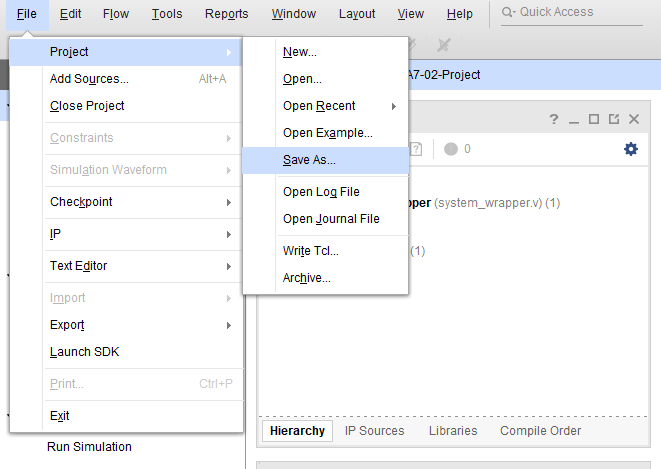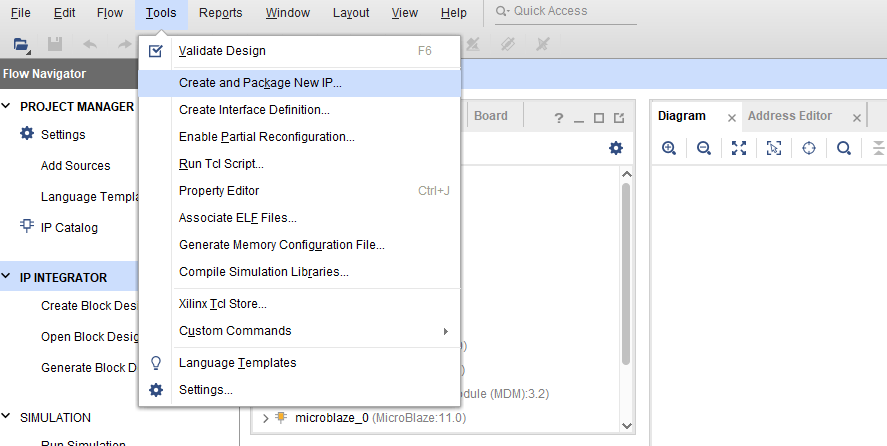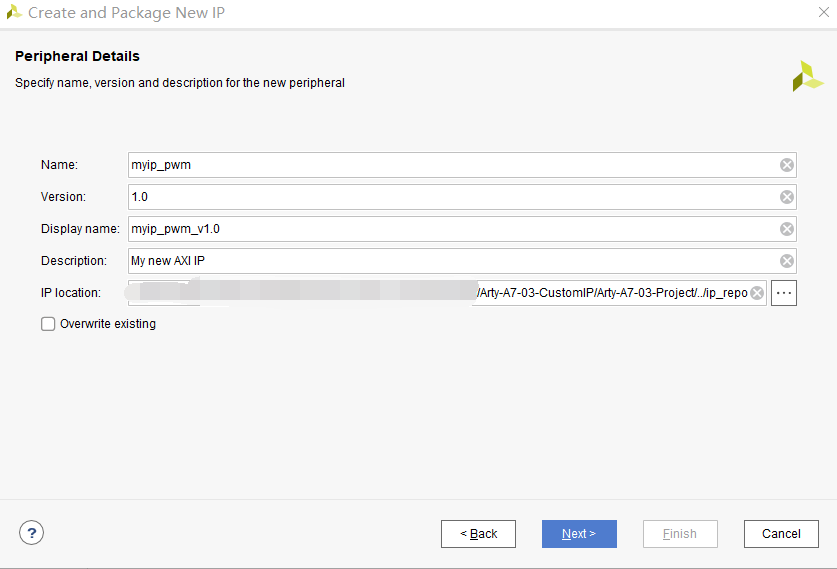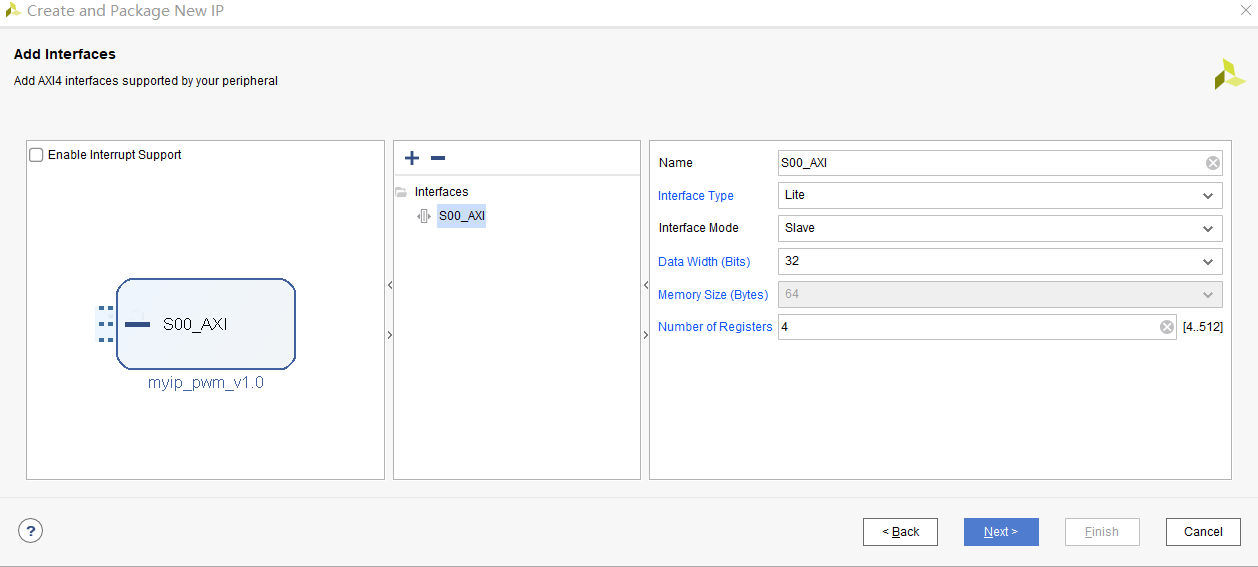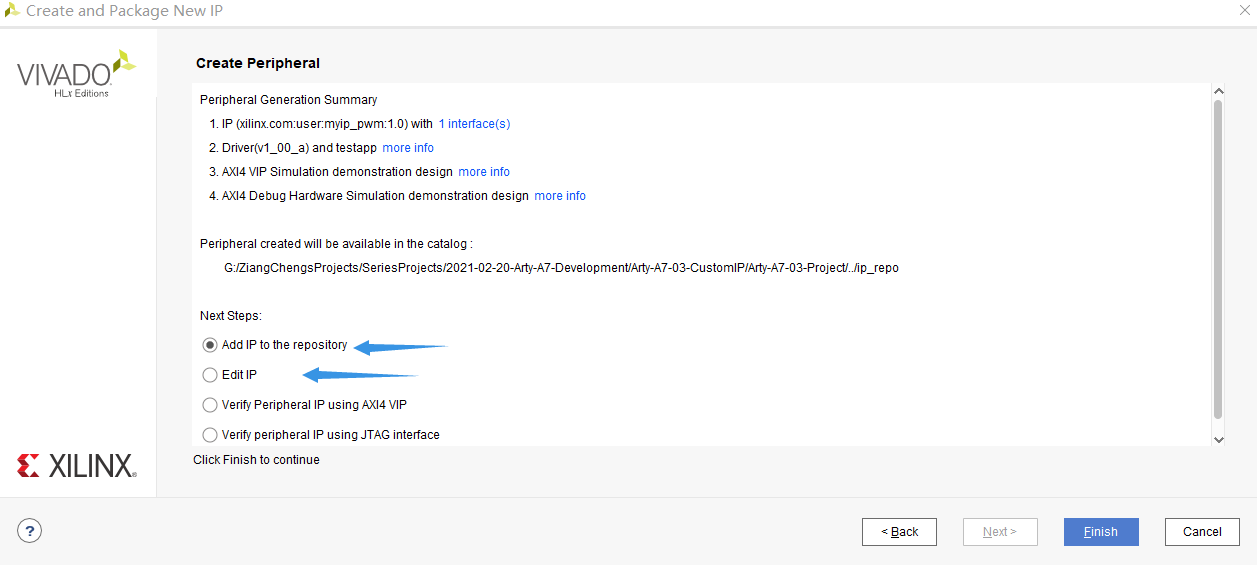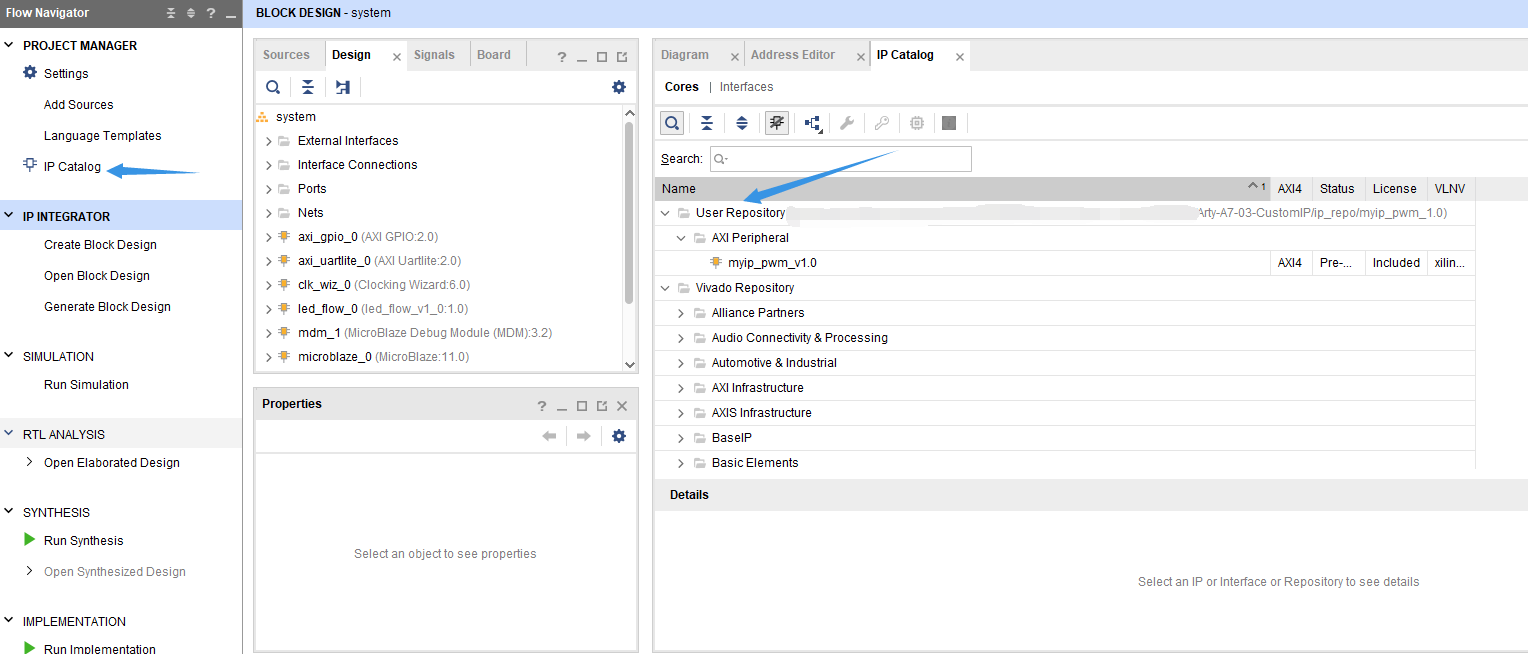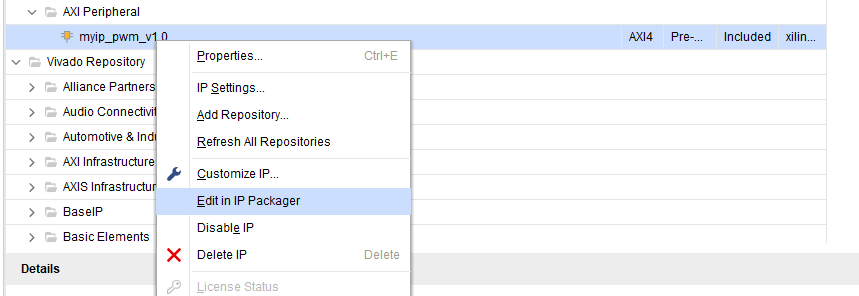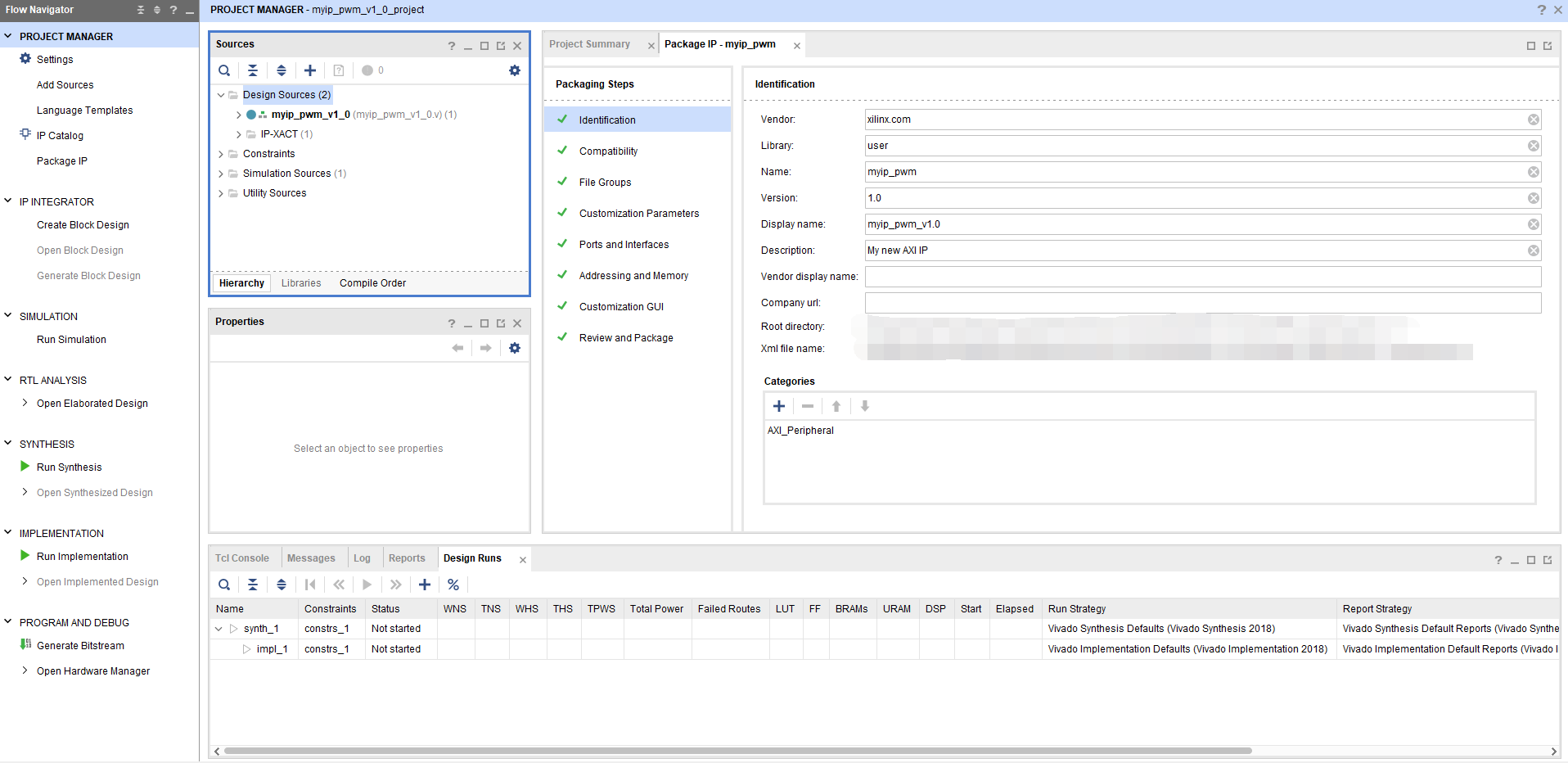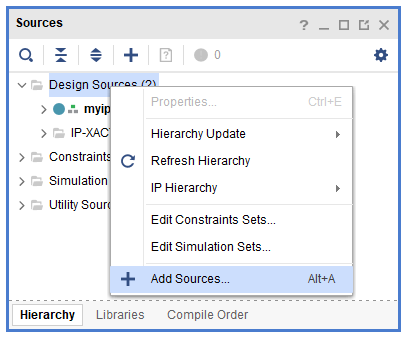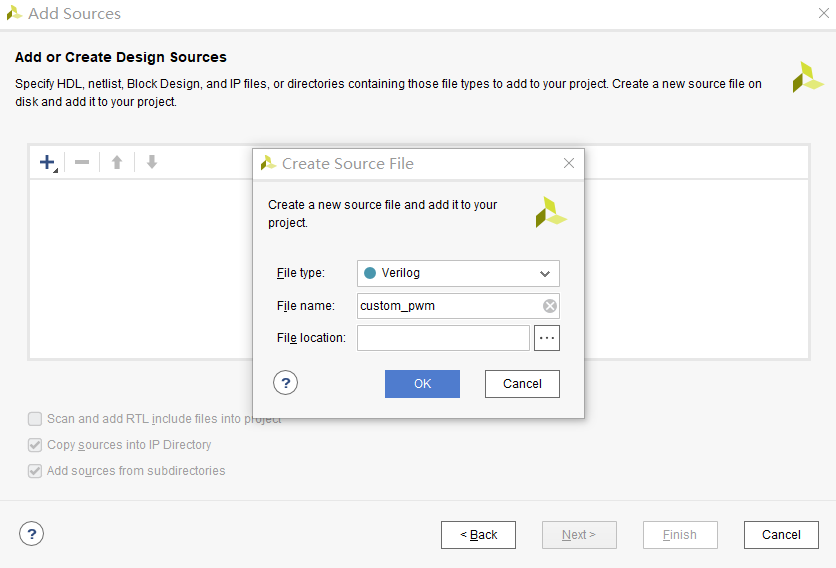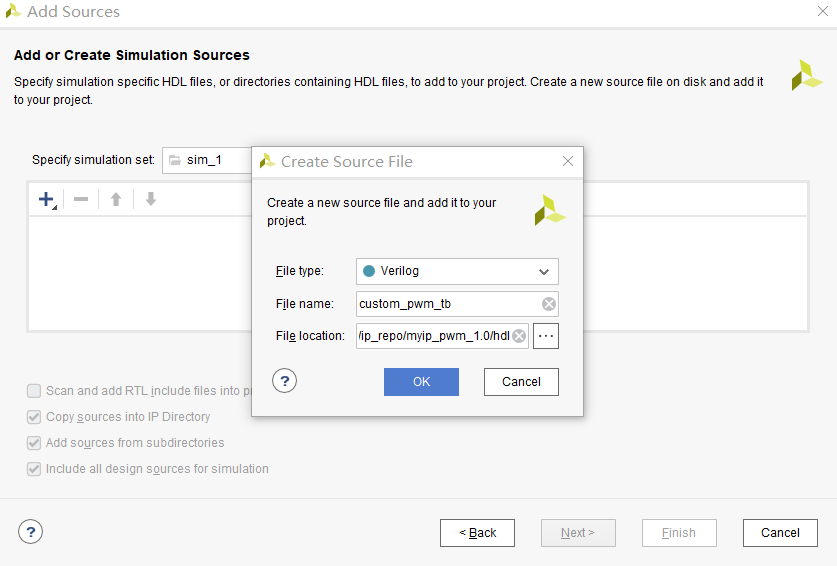custom_pwm.v文件中添加了如下代码。输入接口中，psc用于设置预分频系数，实际上是一个计数器的溢出值，用于将输入时钟分频再驱动PWM的主计数器，以实现更大范围可调的PWM频率；arr用于设计PWM的主计数器溢出值，与psc一同决定PWM频率；cmp用于设定比较值，其将与PWM的主计数器值进行比较，以完成PWM输出，实际上这个输入决定了PWM的占空比。

timescale 1ns / 1psPWM

// 注释略

module custom_pwm (
input           clk,
input           rst_n,
input           w_en,
input  [15:0]   psc,
input  [15:0]   arr,
input  [15:0]   cmp,
output          pwm
);

// Regist inputs
reg  [15:0] psc_reg;    // Prescale register
reg  [15:0] arr_reg;    // Accumulate register
reg  [15:0] cmp_reg;    // Compare register

always @(posedge clk) begin
if (!rst_n) begin
psc_reg <= 16'd0;
arr_reg <= 16'd0;
cmp_reg <= 16'd0;
end else begin
if (w_en) begin
psc_reg <= psc;
arr_reg <= arr;
cmp_reg <= ccr;
end else begin
psc_reg <= psc_reg;
arr_reg <= arr_reg;
cmp_reg <= cmp_reg;
end
end
end

// Prescale
reg  [15:0] psc_cnt;
wire        psc_out;
always @(posedge clk) begin
if (!rst_n) begin
psc_cnt <= 16'd0;
end else begin
if (psc_cnt < psc_reg - 1) begin
psc_cnt <= psc_cnt + 1'b1;
end else begin
psc_cnt <= 16'd0;
end
end
end
assign psc_out = (psc_cnt == psc_reg - 1) ? 1'b1 : 1'b0;

reg  [15:0] main_cnt;
always @(posedge clk) begin
if (!rst_n) begin
main_cnt <= 16'd0;
end else begin
if (psc_out) begin
if (main_cnt < arr_reg - 1) begin
main_cnt <= main_cnt + 1'b1;
end else begin
main_cnt <= 16'd0;
end
end else begin
main_cnt <= main_cnt;
end
end
end

// Compare and output
assign pwm = (main_cnt >= cmp_reg) ? 1'b0 : 1'b1;

endmodule


custom_pwm.v文件中添加了如下代码，用于简略测试custom_ip模块，确定它可以实现预期的功能。

timescale 1ns / 1psPWM

// 注释略

module custom_pwm_tb ();

reg         clk;
reg         rst_n;
reg         w_en;
reg  [15:0] psc;
reg  [15:0] arr;
reg  [15:0] cmp;
wire        pwm;

custom_pwm
u_custom_pwm (
.clk    (clk    ),
.rst_n  (rst_n  ),
.w_en   (w_en   ),
.psc    (psc    ),
.arr    (arr    ),
.cmp    (cmp    ),
.pwm    (pwm    )
);

always #10 clk <= ~clk;

initial begin
clk     <= 1'b0;
rst_n   <= 1'b0;
w_en    <= 1'b0;
psc     <= 15'd4;
arr     <= 15'd8;
cmp     <= 15'd1;
#20
rst_n   <= 1'b1;
#30
w_en    <= 1'b1;
#40
w_en    <= 1'b0;
#2000
cmp     <= 15'd7;
#2100
w_en    <= 1'b1;
#2200
w_en    <= 1'b0;
end

endmodule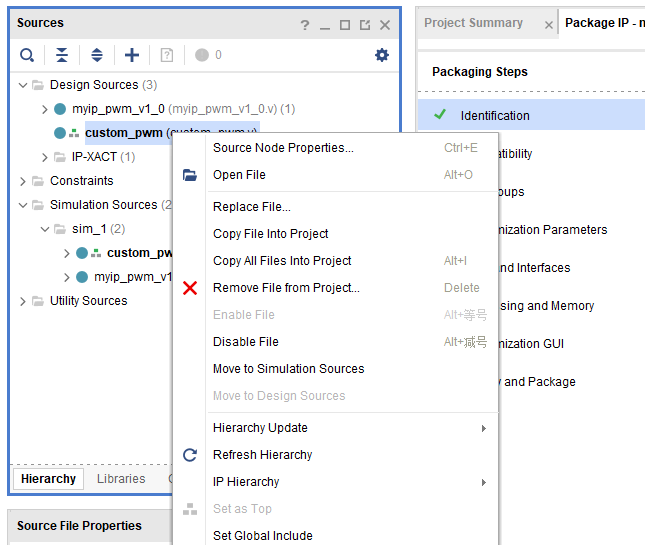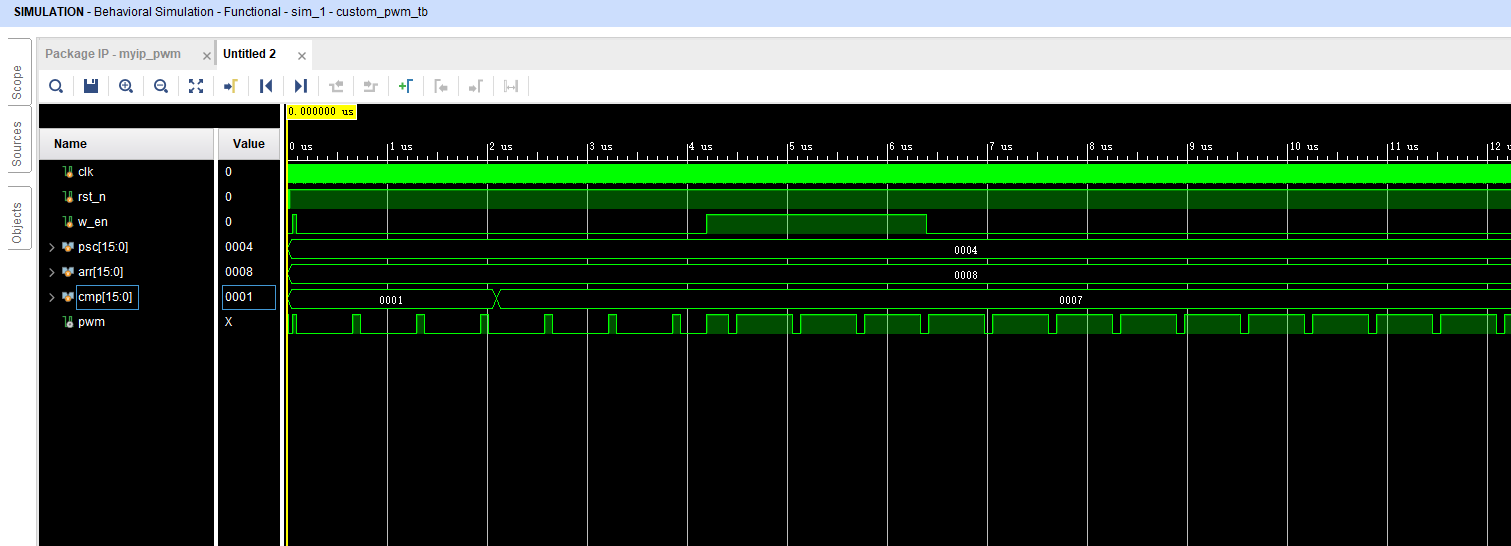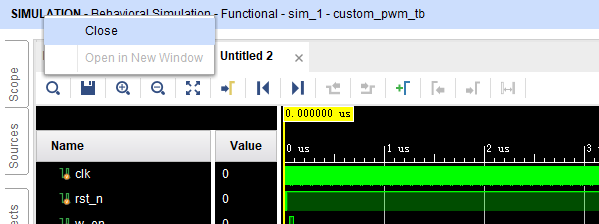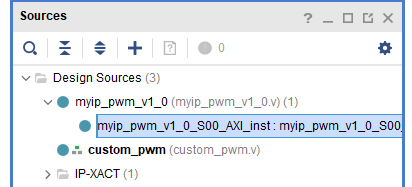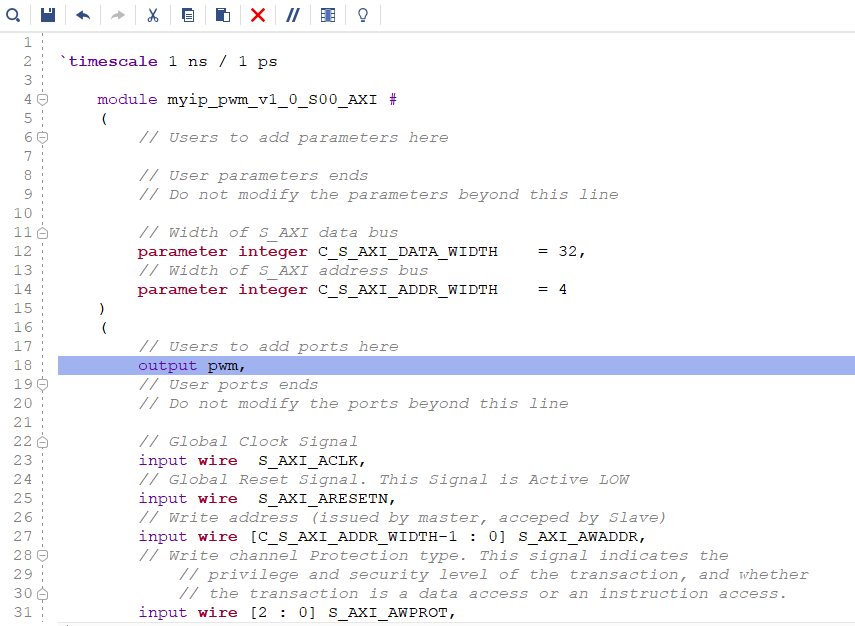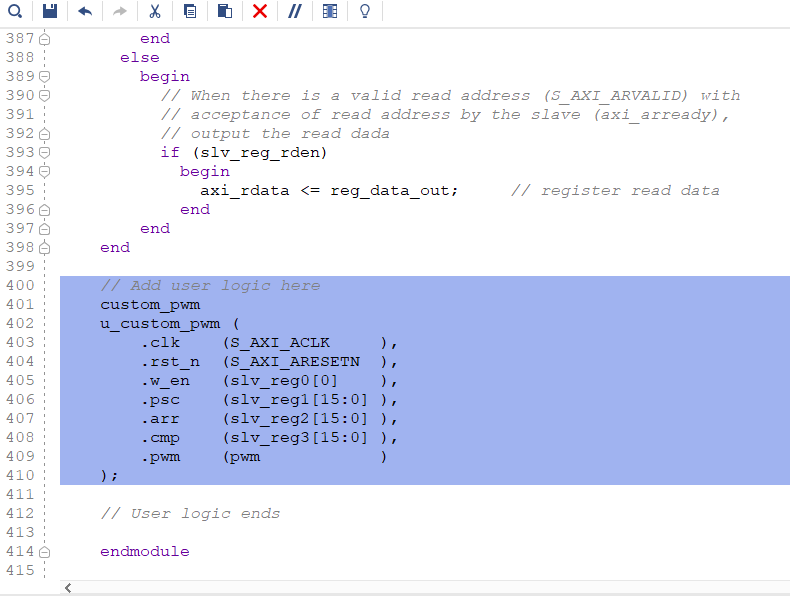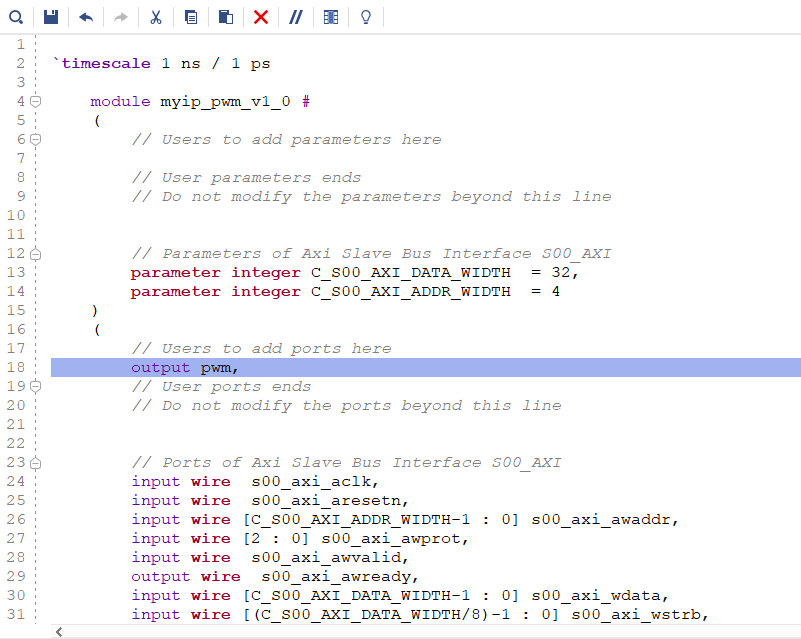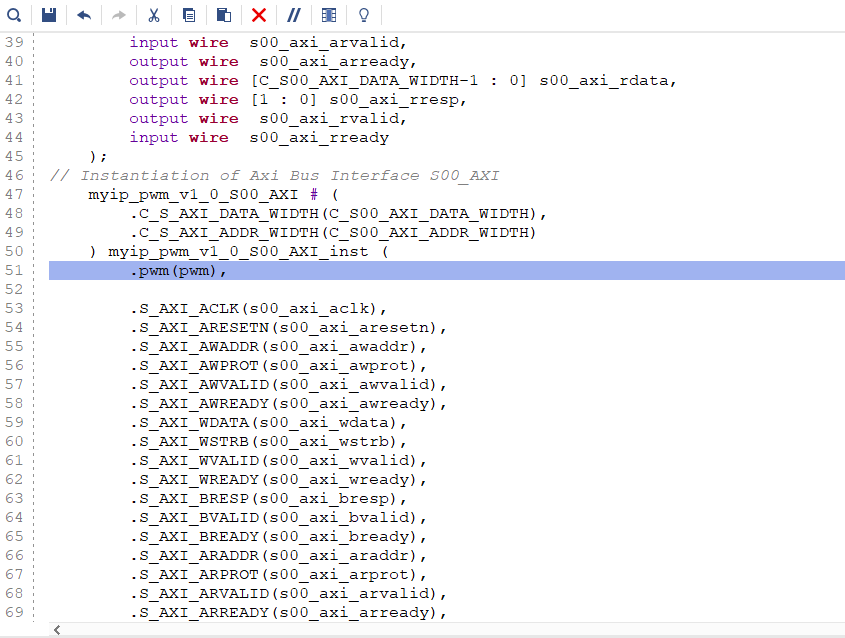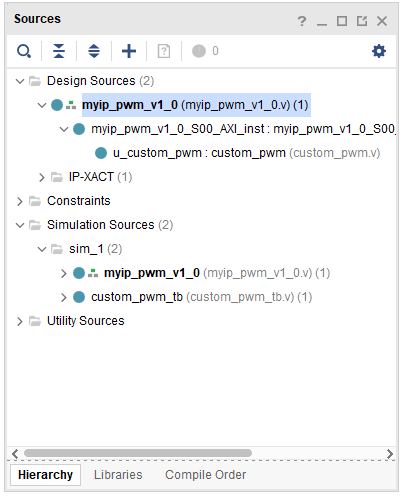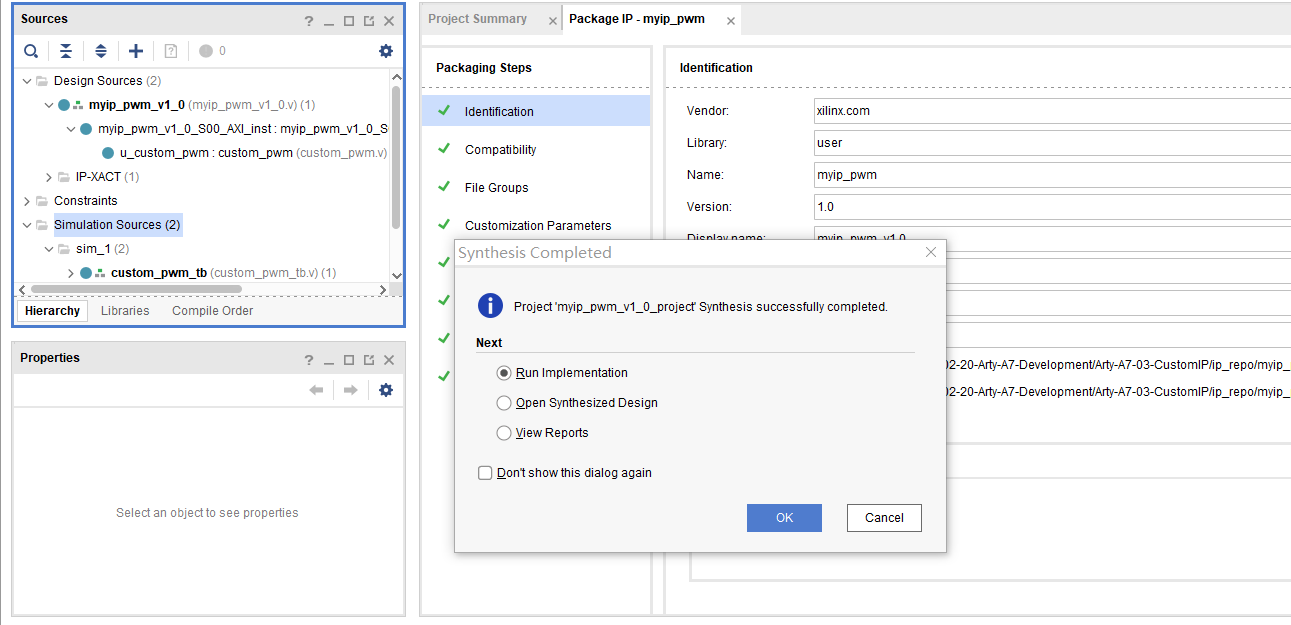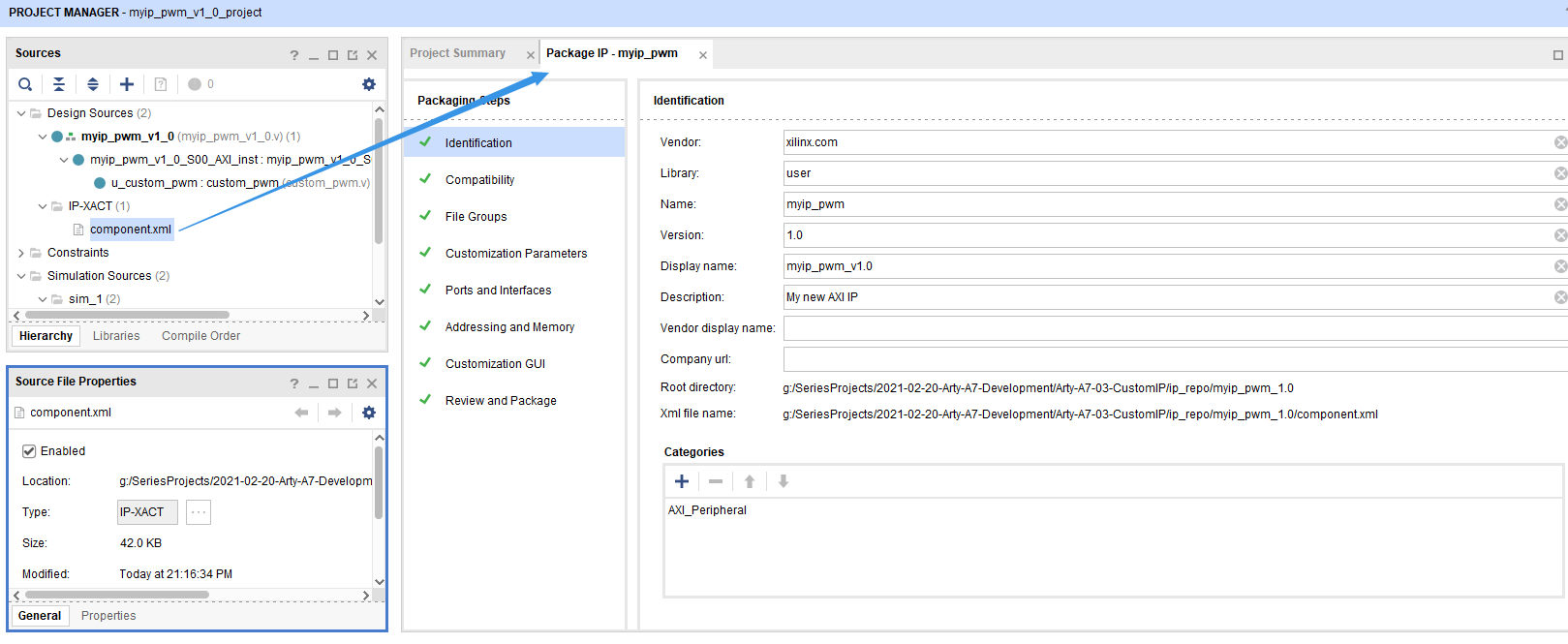File Groups点击Merge changes from File Groups Wizard，这样会重新生成IP的相关文件，注意其中还包含软件驱动代码。这些都位于IP的目录下：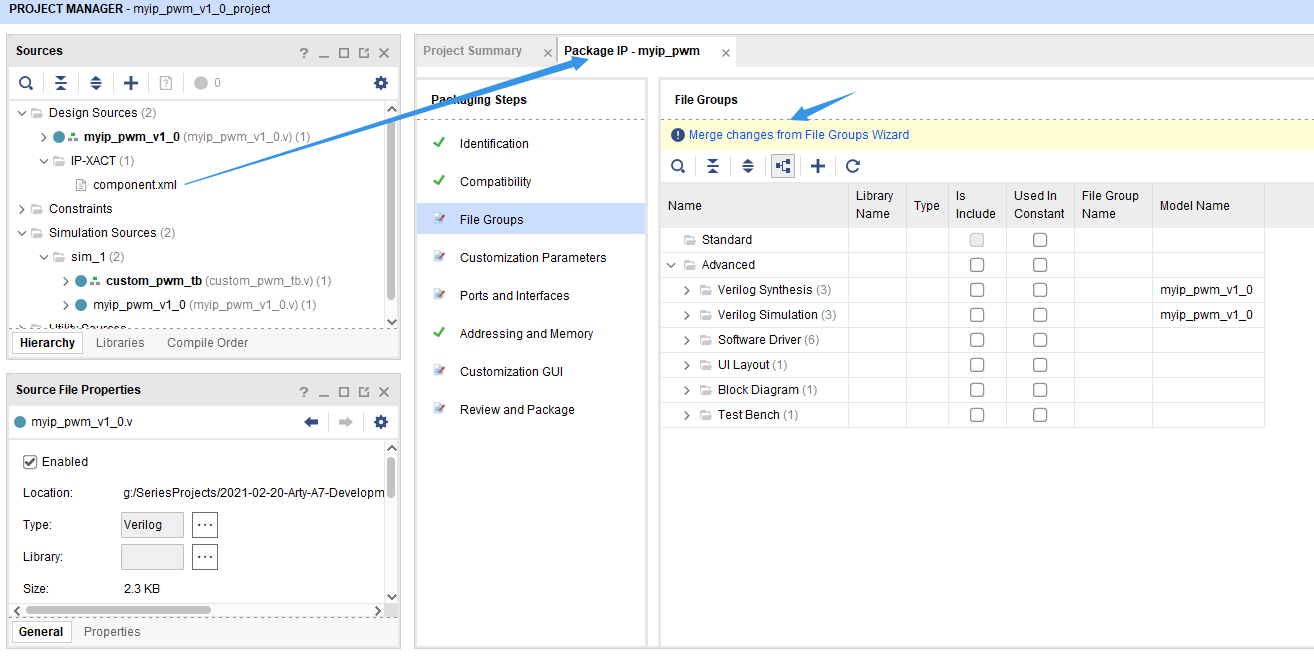GUI界面，可以预览IP在Block Design中的外形，以及调整引脚的排列顺序：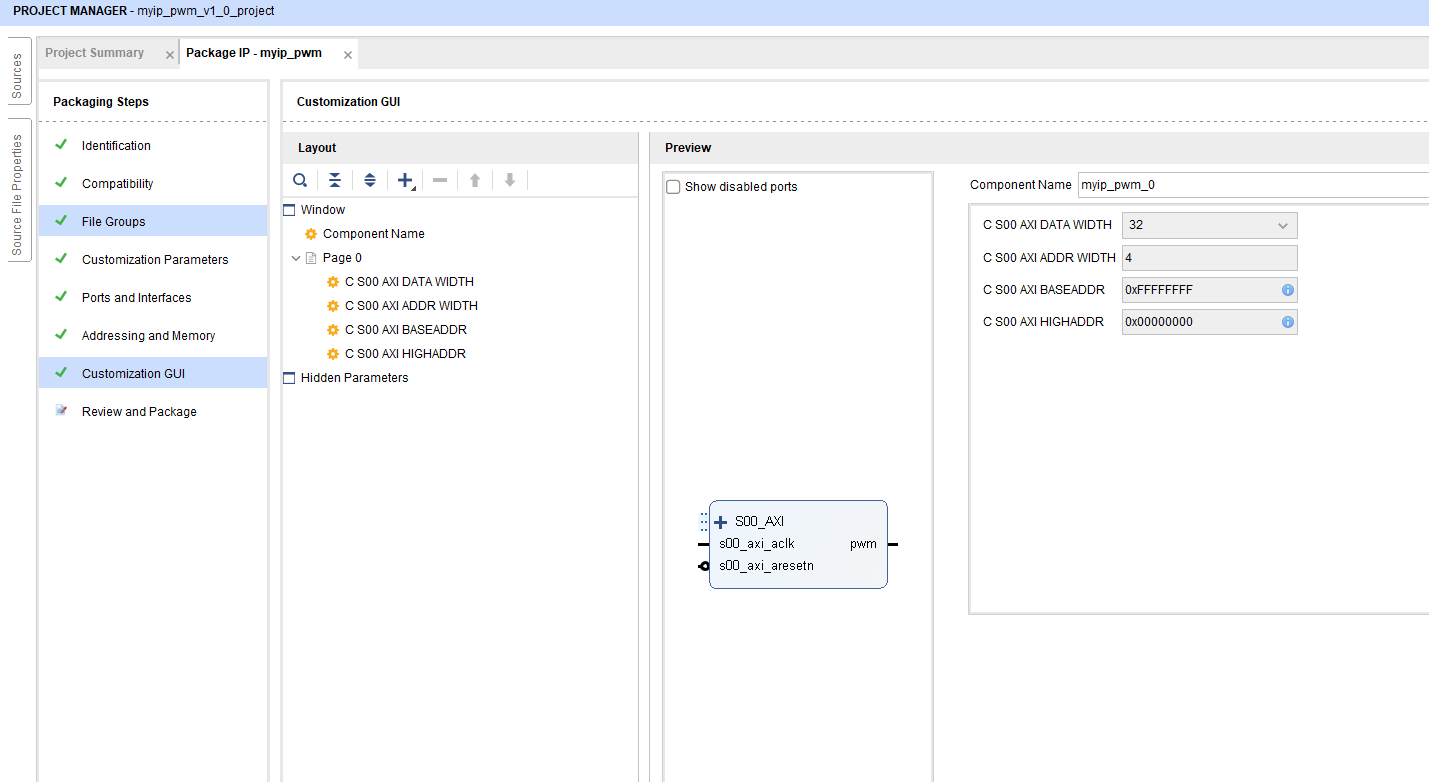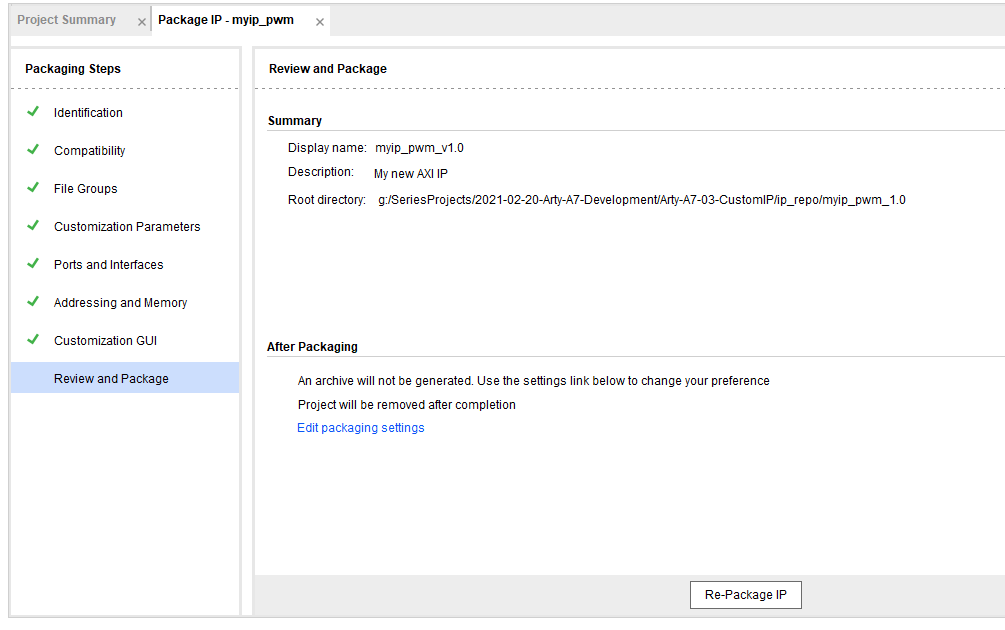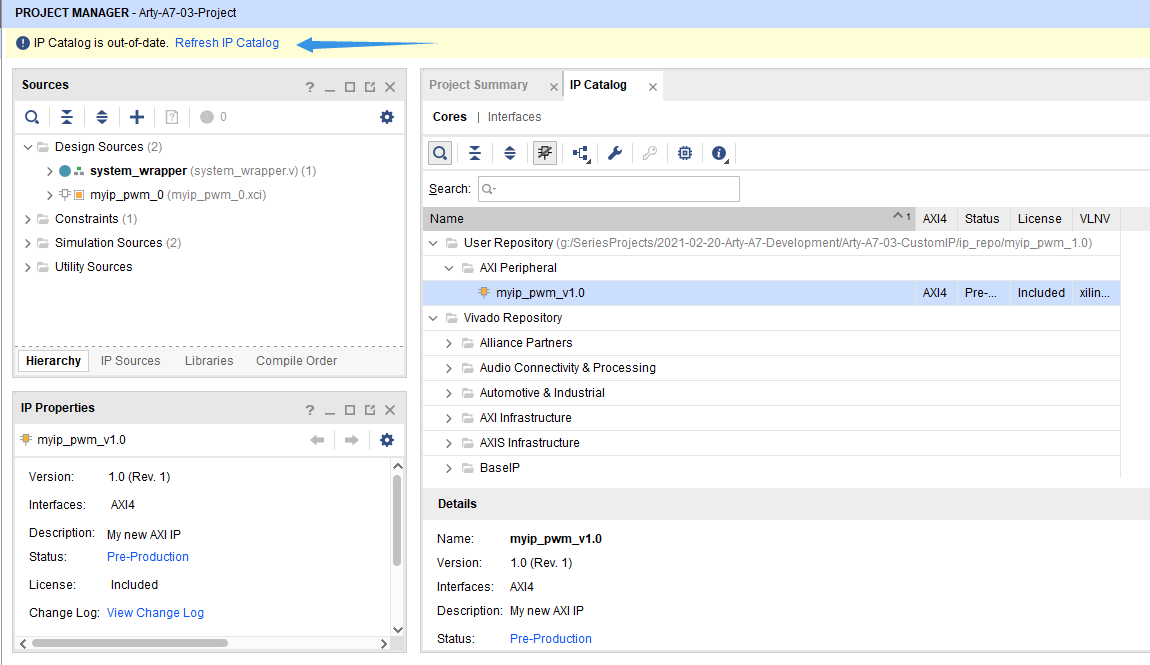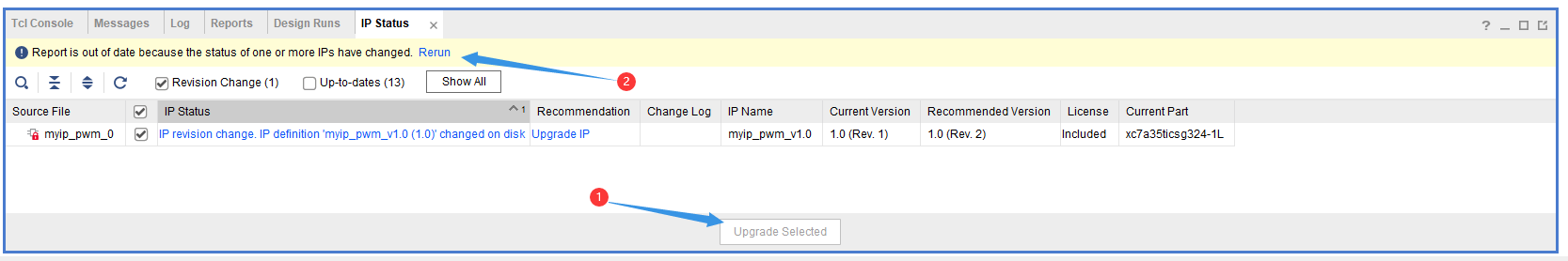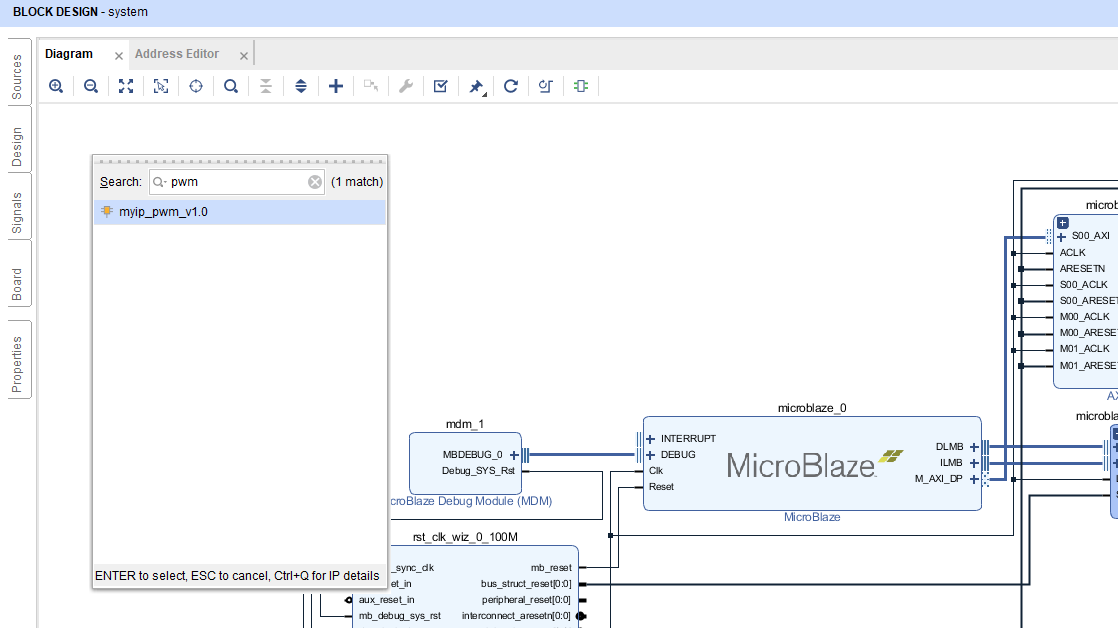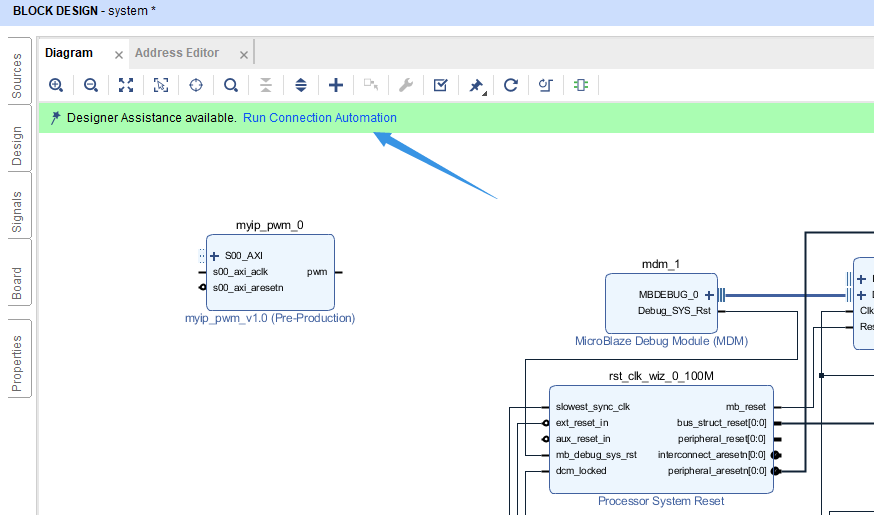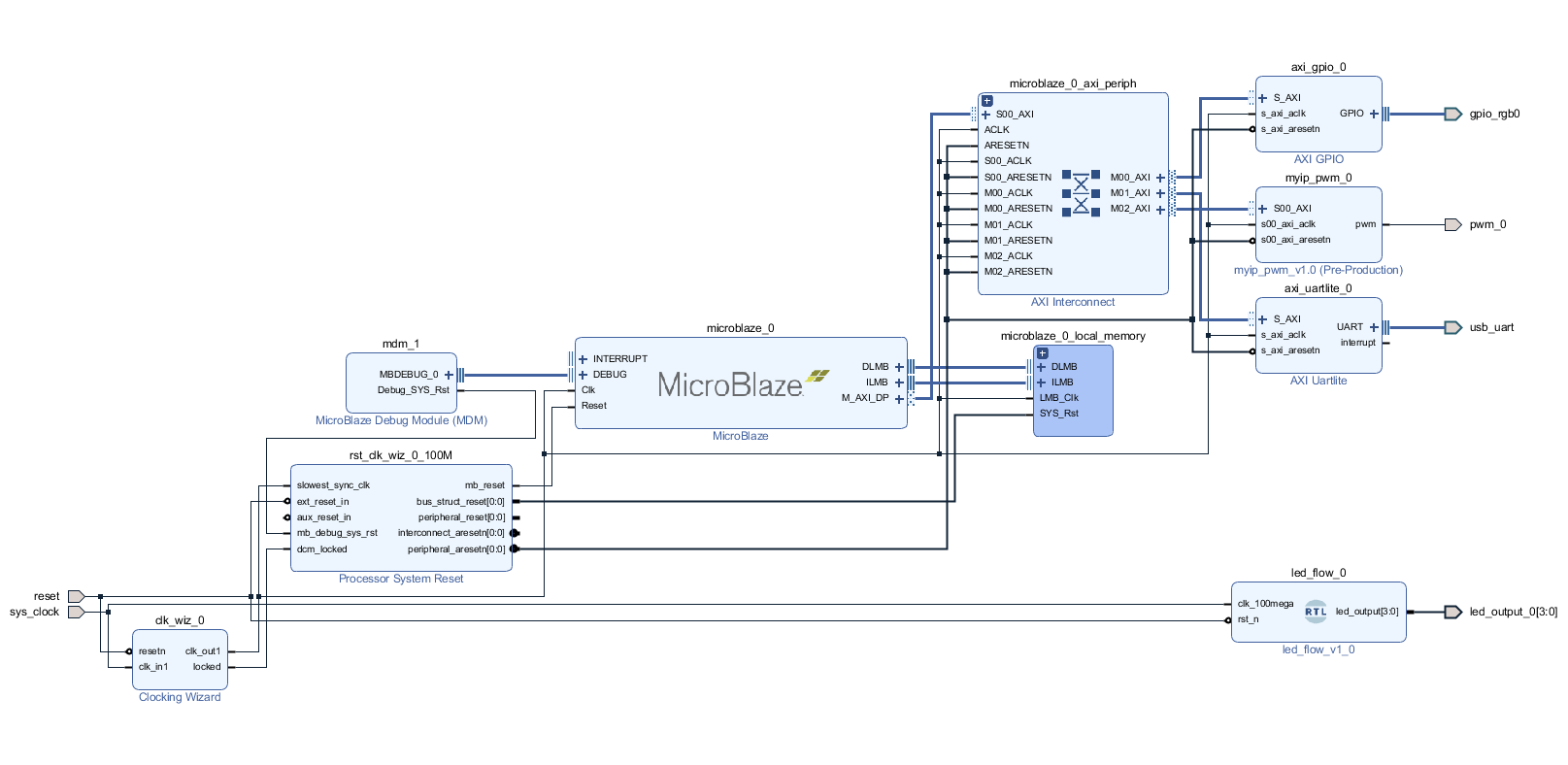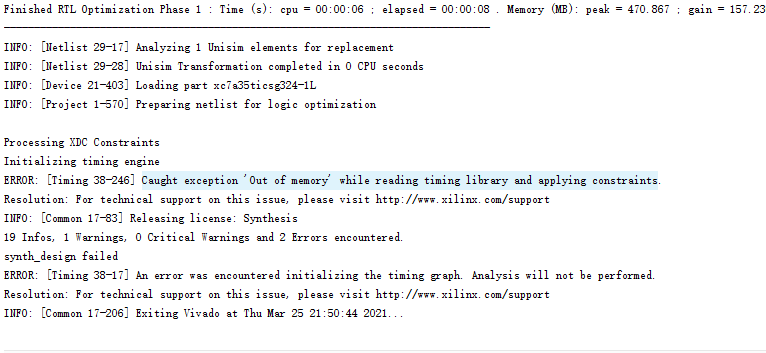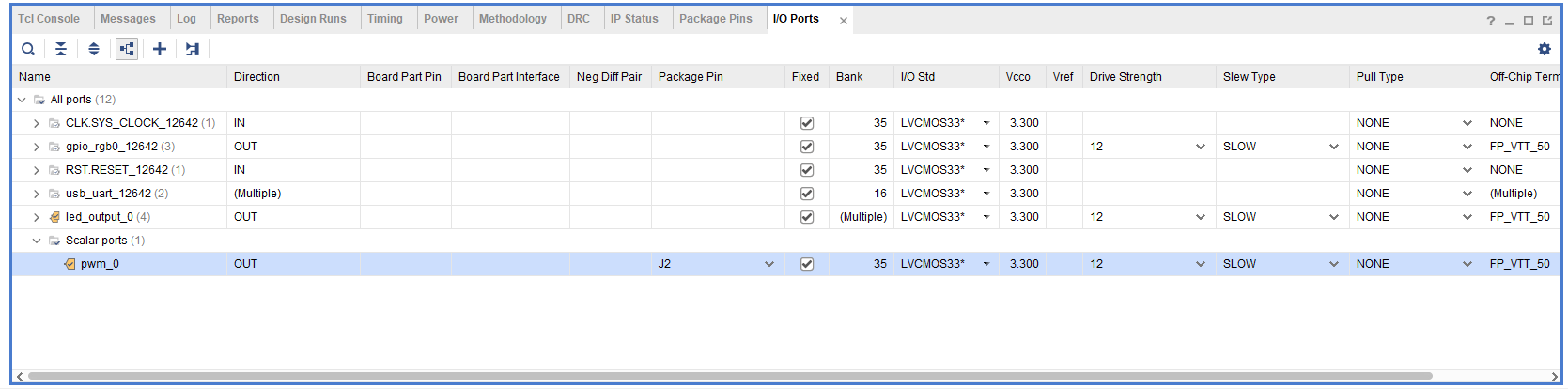### 1.2.软件设计xparameters.h文件中可以看到相关AXI总线地址的定义：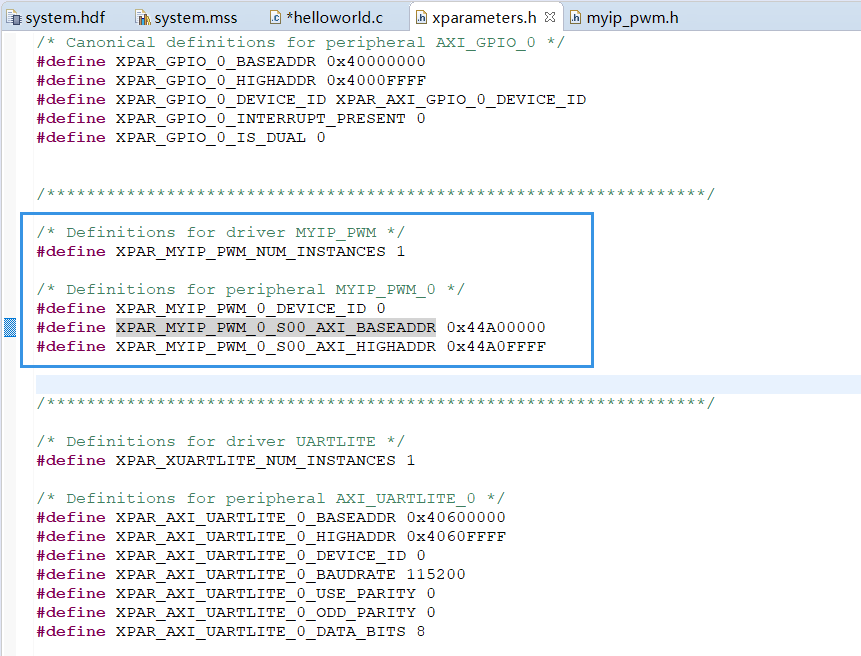myip_pwm.h文件中可以看到四个寄存器的偏移地址定义：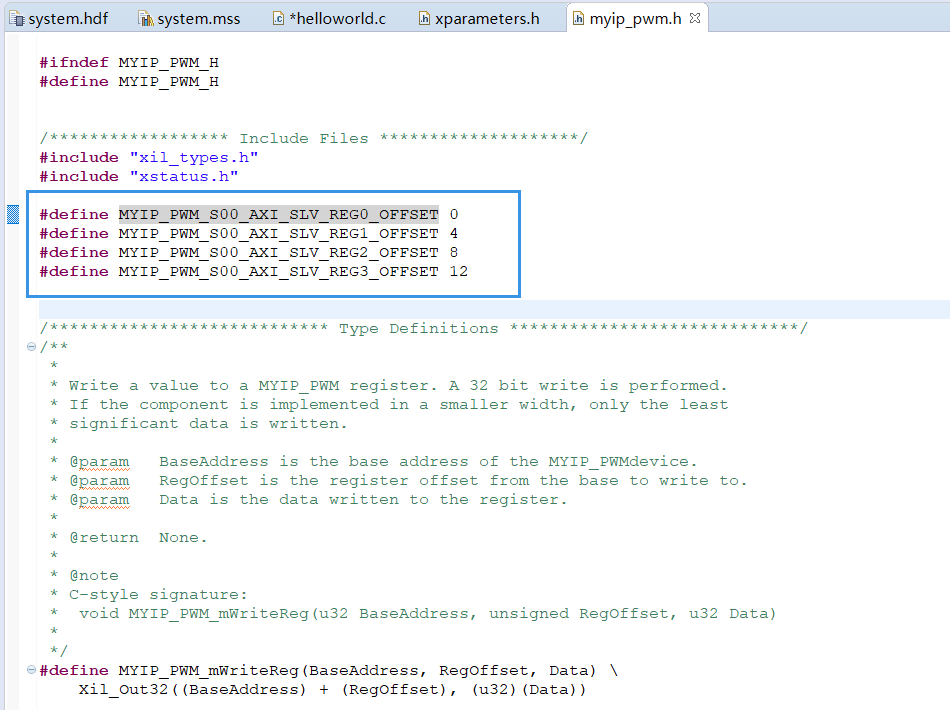helloworld.h中的完整代码如下：

#include
#include "platform.h"
#include "xil_printf.h"

#include "sleep.h"
#include "xparameters.h"
#include "xgpio.h"

#include "myip_pwm.h"

/* RGB LED 0 ----------------------------------------------------------------*/
#define RGB0_ID XPAR_GPIO_0_DEVICE_ID
#define RGB0_CH 1
XGpio Rgb0Gpio;

/* Custom PWM ---------------------------------------------------------------*/
#define PWM_IP_RCFG MYIP_PWM_S00_AXI_SLV_REG0_OFFSET
#define PWM_IP_RPSC MYIP_PWM_S00_AXI_SLV_REG1_OFFSET
#define PWM_IP_RARR MYIP_PWM_S00_AXI_SLV_REG2_OFFSET
#define PWM_IP_RCCR MYIP_PWM_S00_AXI_SLV_REG3_OFFSET

int main() {
uint16_t time_ms;
uint8_t pwm_inc_dir;
uint16_t pwm_duty;

init_platform();
print("Custom IP Test: \n\r");

// RGB0 initialize
XGpio_Initialize(&Rgb0Gpio, RGB0_ID);
XGpio_SetDataDirection(&Rgb0Gpio, RGB0_CH, 0);  // Set as output

// Custom PWM initialize
MYIP_PWM_mWriteReg(PWM_IP_BASE, PWM_IP_RCFG, 0x0001);   // Enable
MYIP_PWM_mWriteReg(PWM_IP_BASE, PWM_IP_RPSC, 100);      // Set prescale to 100
MYIP_PWM_mWriteReg(PWM_IP_BASE, PWM_IP_RARR, 1000);     // Set period to 1000
MYIP_PWM_mWriteReg(PWM_IP_BASE, PWM_IP_RCCR, 500);      // Set duty to 50%

while (1) {
// RGB0 is shifted every 500ms
// PWM duty is updated every 10ms
// (Approximately)
for (time_ms = 0; time_ms < 1500; time_ms += 10) {
// Shift RGB0
XGpio_DiscreteWrite(&Rgb0Gpio, RGB0_CH, 0x01 << (time_ms/500));

// Increase or decrease PWM duty
if (pwm_inc_dir == 0) {
pwm_duty = pwm_duty + 10;
} else {
pwm_duty = pwm_duty - 10;
}
if (pwm_duty == 1000) {
pwm_inc_dir = 1;
} else if (pwm_duty == 0) {
pwm_inc_dir = 0;
}

// Write duty value to custom PMW IP
MYIP_PWM_mWriteReg(PWM_IP_BASE, PWM_IP_RCCR, pwm_duty);

// Delay 10ms
usleep(10*1000);

}
}

cleanup_platform();
return 0;

}